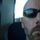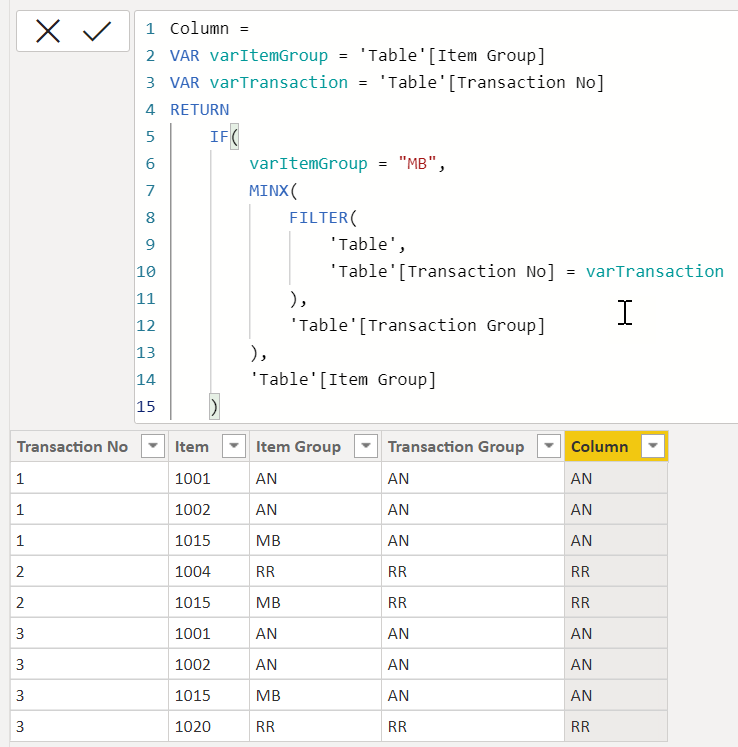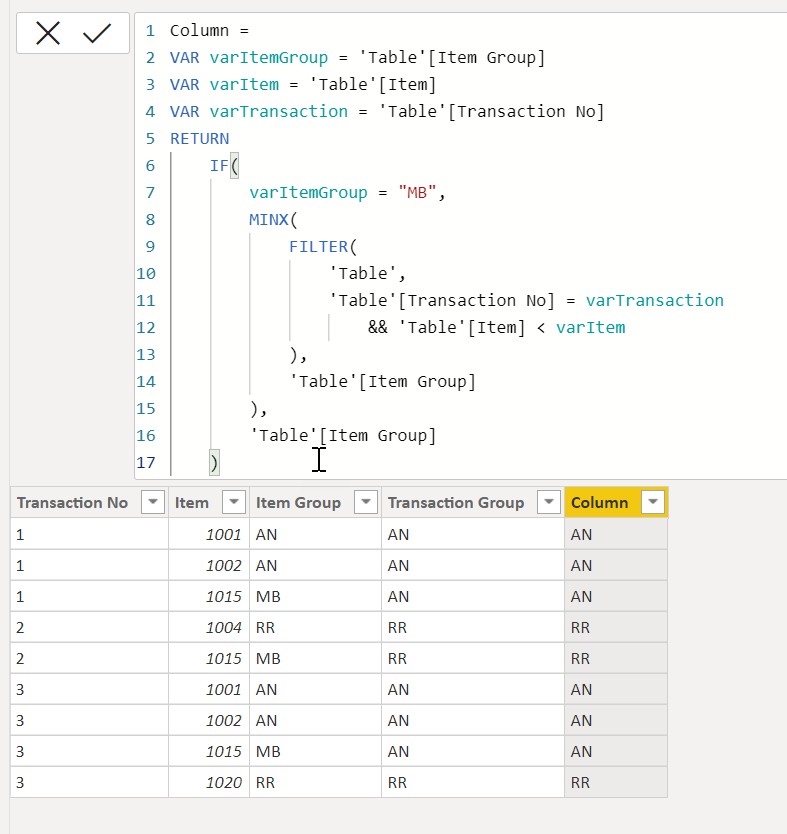cancel
Showing results for
Did you mean:Frequent Visitor

## LOOKUP in SAME TABLE

Hi All,

I would like to ask assistance in the scenario below:

currently have a table with the first three columns, and I want to add a column for the Transaction Group.
Rules:

> If Item group is MB, get the first item group with same transaction no.
> If item is not MB, get row item group.

 Transaction No Item Item Group Transaction Group 1 1001 AN AN 1 1002 AN AN 1 1015 MB AN 2 1004 RR RR 2 1015 MB RR 3 1001 AN AN 3 1002 AN AN 3 1015 MB AN 3 1020 RR RR

Thank you.

1 ACCEPTED SOLUTIONFrequent Visitor

Hi Greg, this worked for my sample dataset. will check on the full dataset before accepting as solution. thank you.

6 REPLIES 6Super User

Is this what you want?You said you wanted the "row item group" if Item Group wasn't MB, but I wasn't sure what that meant. I pulled the item group from the same row.

Code here:

``````Column =
VAR varItemGroup = 'Table'[Item Group]
VAR varTransaction = 'Table'[Transaction No]
RETURN
IF(
varItemGroup = "MB",
MINX(
FILTER(
'Table',
'Table'[Transaction No] = varTransaction
),
'Table'[Transaction Group]
),
'Table'[Item Group]
)``````

Note: This is a calculated column formula and must be entered as a New Column to work.

Did my answers help arrive at a solution? Give it a kudos by clicking the Thumbs Up!

DAX is for Analysis. Power Query is for Data Modeling

Proud to be a Super User!

MCSA: BI ReportingFrequent Visitor

Hi. noticed that you used the transaction group column in the formula. What i meant was that, I am trying to have the trans group column be the output.

Thank you.Super User

Ahh.. DIdn't understand TransactionGroup was the expected result. Try this @MSanchezz

``````Column =
VAR varItemGroup = 'Table'[Item Group]
VAR varItem = 'Table'[Item]
VAR varTransaction = 'Table'[Transaction No]
RETURN
IF(
varItemGroup = "MB",
MINX(
FILTER(
'Table',
'Table'[Transaction No] = varTransaction
&& 'Table'[Item] < varItem
),
'Table'[Item Group]
),
'Table'[Item Group]
)``````Did my answers help arrive at a solution? Give it a kudos by clicking the Thumbs Up!

DAX is for Analysis. Power Query is for Data Modeling

Proud to be a Super User!

MCSA: BI ReportingSuper User

@MSanchezz I think I got it correct, please check, I did not use Transaction Group in my formula.

Become an expert!: Enterprise DNA
External Tools: MSHGQM
Latest book!:
Mastering Power BI 2nd EditionDAX is easy, CALCULATE makes DAX hard...Super User

@MSanchezz Maybe:

``````Transaction Group =
IF(
"Item Group" = "MB",
VAR __First = MINX(FILTER('Table',[Transaction]=EARLIER([Transaction])),[Item])
RETURN
MAXX(FILTER('Table',[Transaction]=EARLIER([Transaction]) && [Item]=__First),[Item Group]),
[Item Group]
)``````

Become an expert!: Enterprise DNA
External Tools: MSHGQM
Latest book!:
Mastering Power BI 2nd EditionDAX is easy, CALCULATE makes DAX hard...Frequent Visitor

Hi Greg, this worked for my sample dataset. will check on the full dataset before accepting as solution. thank you.